Geometry

# Angles and Lines: Level 3 Challenges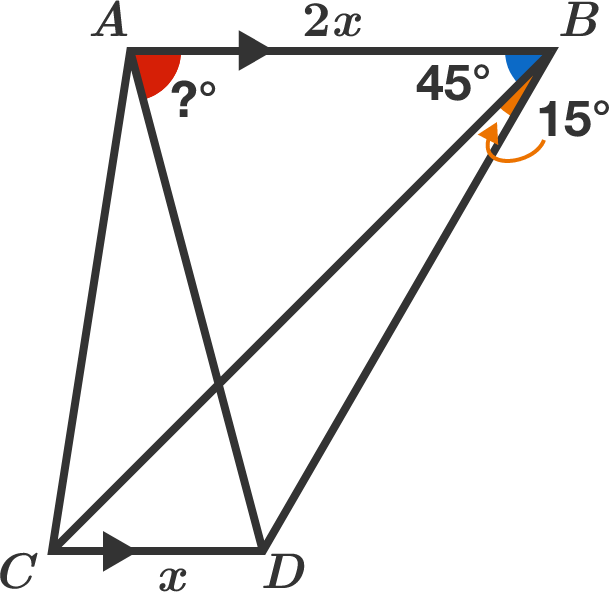$AB$ is parallel to $CD$ and $AB =2CD$.

If $m\angle ABC = 45^o$ and $m\angle CBD = 15^o,$ find $m\angle BAD$ in degrees.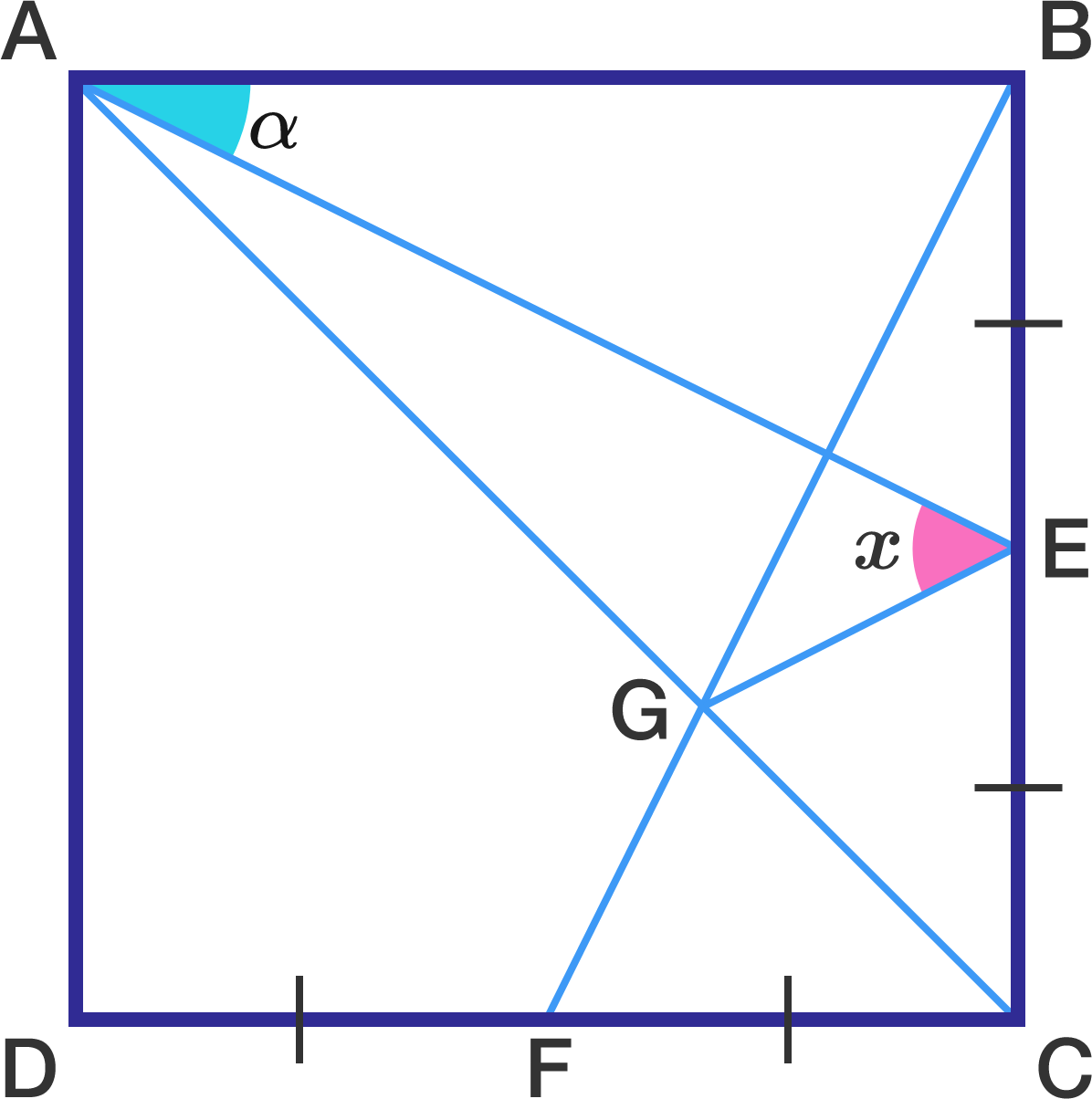In the square above, $E$ and $F$ are the midpoints of $\overline{BC}$ and $\overline{CD} ,$ respectively. If $G$ is the intersection point of $\overline{AC}$ and $\overline{BF}$ and $x=\angle AEG,$ find $x$ (in degrees) in terms of $\alpha.$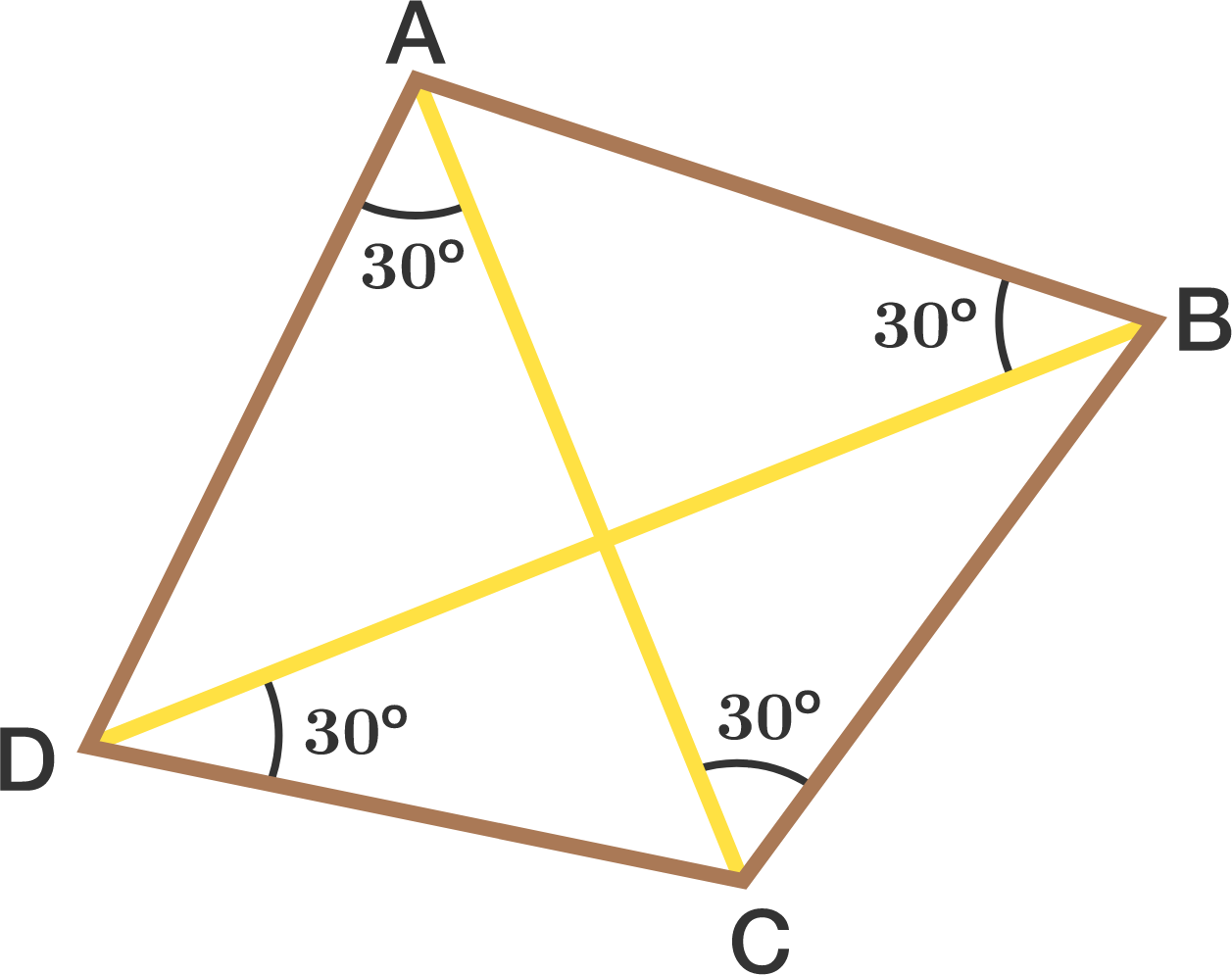In a quadrilateral $ABCD$, $\angle ABD = \angle BCA = \angle CDB = \angle DAC = 30^\circ$.

Find the smaller of the two angles (in degrees) between the diagonals AC and BD.


Note: The image might not be necessarily up to scale.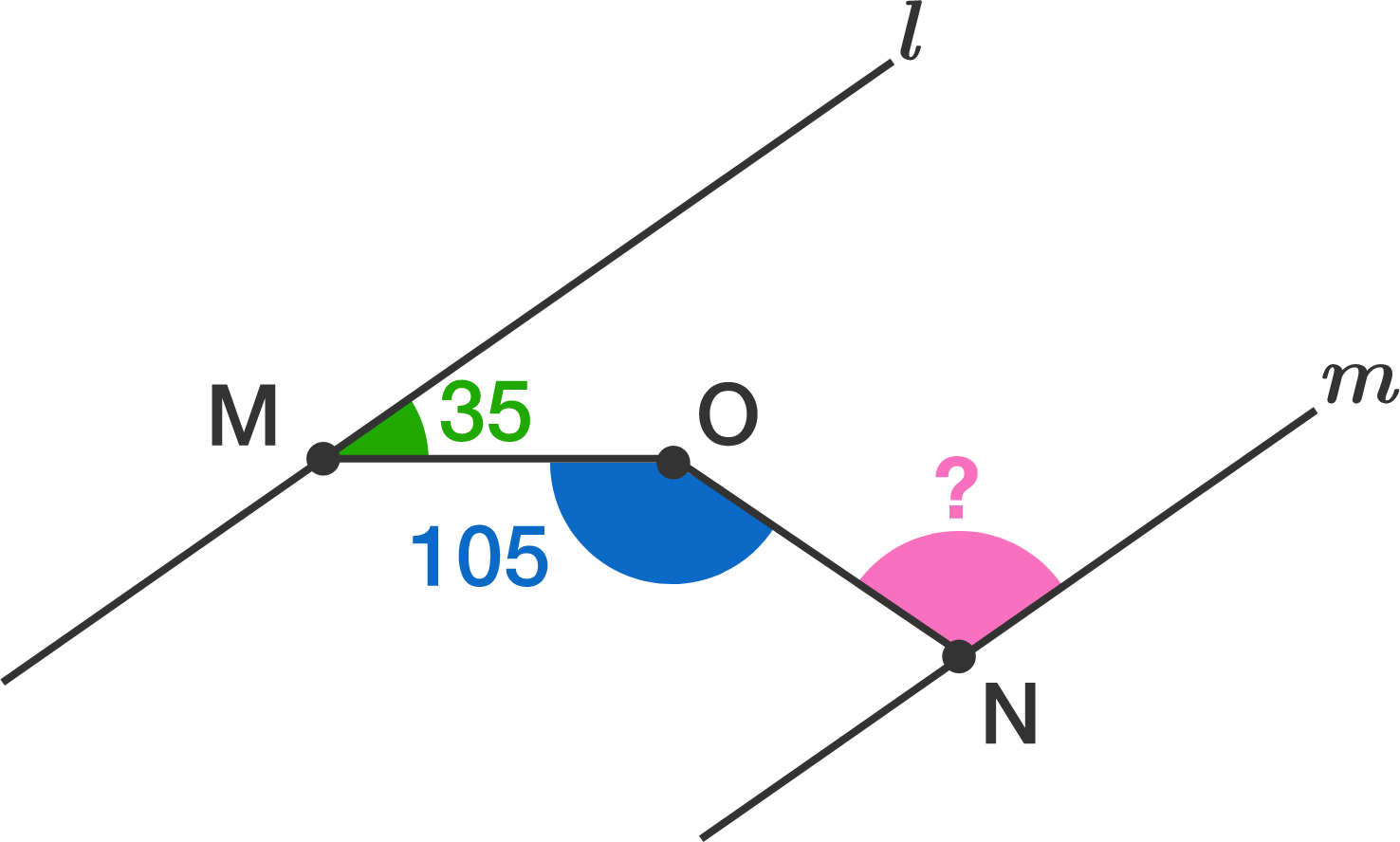Lines $l$ and $m$ are parallel. $M$, $N$ and $O$ are points such that $M$ is on $l$ and $N$ is on $m$ and $O$ is between the two lines. If the smaller angle between $l$ and $MO$ is $35^{\circ}$ and $\angle MON = 105^{\circ}$, find the acute angle between $m$ and $NO$.

Clarification: The diagram is not accurate

Find $x$ (in degrees).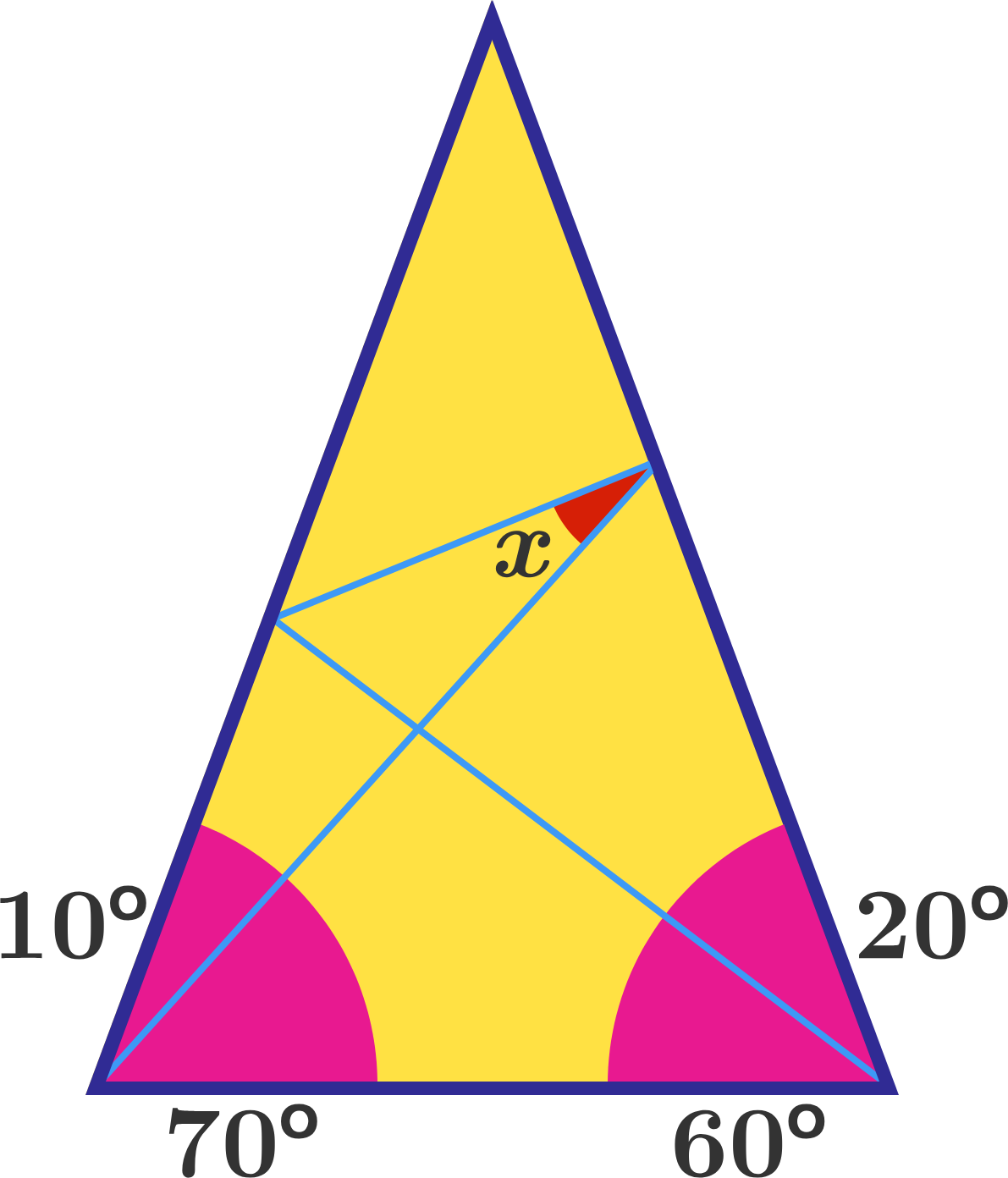×